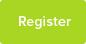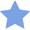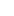# Tutor Hunt Questions

1. If you write all the numbers from 1 to 100, how many times will the digit 9 appear?

2. How many spots are there altogether on five, normal, six-sided dice?

3. How can you use six nines to make 100?
3 years ago

Please enter your response to the question below. The student will get a notification as soon your response has been approved by our moderation team.

####Accepted Answer

1. 20 times. 9 19 29 39 49 59 69 79 89 90, 91, 92, 93,...,99 . technically if you do not specify whole numbers then infinity.
2. 21 * 5 = 105
3. (9 * 9) + 9 + 9 + (9 / 9) = 100
1. 20
2. 105
3. (999 - 99) / 9 = 100
Answered by Thomas | 3 years ago
We can use simple symmetries instead of brutal force to solve the problems.

Problem 1.
Observe: 00, 01, 02, 03, 04, ... 09, 10, 11, ... 99
The number of appearances for 0 to 9 are symmetric therefore dight 9 appears 2*100/10=20 times

Problem 2.
5*(1+2+3+4+5)=105

Problem 3.
9+9/9=10, therefore (9+9/9)(9+9/9)=100

Thanks
Answered by Jett | 3 years ago
1) 11 nines will appear.

2) 105 dots will appear.

3) 9x9=81+9+9=99+(9/9)=100
Answered by Numan | 3 years ago
A) the digit 9 will appear once on its own
B) 105 as there is 21 on 1 so multiply by 5
C) 9 times 9 = 81
81 + 9 = 90
9 divided 9 = 1
1 + 9 = 10
90 + 10 = 100
Answered by Alex | 3 years ago